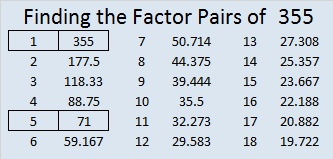# 355 and Level 5

Five can evenly divide into numbers when the last digit is 5, so 355 is a composite number. Scroll down past the puzzle to see its factors.Print the puzzles or type the factors on this excel file: 12 Factors 2015-01-12

• 355 is a composite number.
• Prime factorization: 355 = 5 x 71
• The exponents in the prime factorization are 1 and 1. Adding one to each and multiplying we get (1 + 1)(1 + 1) = 2 x 2 = 4. Therefore 355 has exactly 4 factors.
• Factors of 355: 1, 5, 71, 355
• Factor pairs: 355 = 1 x 355 or 5 x 71
• 355 has no square factors that allow its square root to be simplified. √355 ≈ 18.841This site uses Akismet to reduce spam. Learn how your comment data is processed.